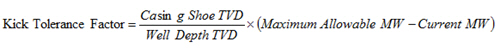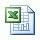# Kick Tolerance Factor Calculation

The following formula is the kick tolerance factor.Where;

Casing shoe TVD in ft

Well depth TVD in ft

Maximum Allowable MW in ppg

Current MW in ppg

For more understanding, let’s try to calculate the kick tolerance factor (KTF) using the following information:

Casing shoe TVD = 5000 ft

Well depth TVD = 11,000 ft

Maximum Allowable MW = 12.9 ppg

Current MW =9.8 ppg

According to the equation above, you will be able to calculate like this;Kick Tolerance Factor =1.41 ppg

Please find the calculation sheet >>Kick Tolerance Factor Calculation

Share the joy1.gas certificate says: